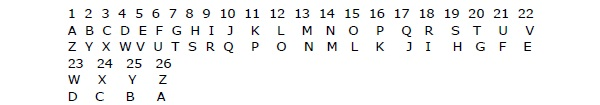# Reasoning - Series Test

A series is a continuous sequence of numbers, letters, or words that are obtained by some particular previously defined rule. Applying that predefined rule, it is possible to find out the next term of the series.

Here we will be discussing series of letters with repeating and non-repeating letters, numbers, letter-number etc.

## Types of Letter Series

There are three types of letter series that we will discuss. They are −

• Letter Series for Repeating Letters
• Letter Series for Non-Repeating Letters
• Letter Series Having Group of Letters

### Letter Series for Repeating Letters

In this type of questions, a group of letters, usually given a small letters, are repeated in a systematic way and thus a series is established. Some cases are presented below −

A sequence of letters in a series may be formed by simply repeating the same group of letters, skipping one letter in turn, in cyclic order.

For Example

Question − b-bca-a-ab

A - abbca

B - babac

C - accbc

D - bbabb

Explanation − If the letters formatting 3 in groups are placed in the blanks respectively, we get a b c b c a c a b a b c. In cyclic order, from a we have abc, from b we have bca and from c cba.

A series of letters may be formed on the basis of some rhythms.

For Example

Question − b-ba-b-b-a-b

A - aabba

B - abaab

C - abbab

D - bbabb

Explanation − If we put the letters of group (4) in the blanks, we get the series − abbabbabbabb- which produces a sort of rhythm.

A sequence of letters may be formed by repeating the same group of letters, skipping one and repeating another.

For Example

Question − ab-c-c-a-ab-a-cc

A - bbcca

B - aabcba

C - cbacbb

D - baccbb

Explanation − If we put the letters of group (3) in the gaps of the series, we obtain abcc bcaa cabb abcc. Here in the group of letters abcc, c is repeated. Next a is dropped from abc. We start from b and last letter in the cycle is repeated

### Letter Series for Non-Repeating Letters

In this type of questions, a series of letters is given, usually in bold letters, where the letters do not repeat. Rather there is a definite rule on the basis of which successive letters are formed.

For Example

Each next letter may be formed by skipping one letter or may be formed by skipping two letters or it may be formed by going two letters back.

Though there is no definite rule or principle in completion of orders of alphabets or letters in a series, yet each question carries a definite pattern or sequence. Certain hints below should be kept in mind.

Keep in mind that the order of letters with their respective numbers, i.e. A-1 to Z-26 and vice versa Z-1 to A-26 as given in the below chart.If you come across a group of questions of letter series type, quickly write down the alphabets and number the letters as above.

For Example

What will come next in the place of the question mark (?) in the following questions?

I - A C F J O ?

II - A D H M S ?

III - D F I M R ?

Explanation

For I − U. Look at letters A, C F, J and O on the numbered alphabet. You will see the pattern: A (1 letter is skipped) C (2 letters are skipped) F (3 letters are skipped) J (4 letters are skipped) O. Therefore the next skip should be 5 letters, bringing to the answer U.

For II − Z. The skipping pattern is 2, 3, 4, 5 and 6 letters.

For III − X. The skipping pattern is 1, 2, 3, 4 and 5 letters.

In the above examples, letters are skipped in increasing order.

### Series Having Group of Letters

In this series, the position of various letters matters a lot.

For Example

Analyze the series and choose the answer that will come in the place of question mark.

AK, FP, ?, PZ, UE, ZJ

A - KU

B - JT

C - JU

D - KV

Explanation − If we write the position numbers of each letter group we will get the following series −

(1, 11), (6, 16), ?, (16, 26), (21, 5), (26, 10).

We see that the first numbers of every pair from a definite series −

1, 6, ?, 16, 21, 26 − where each successive number is obtained by adding 5 to previous number. So the first number in the unknown pair is 11. Consequently the second number of the pair would be 11 + 10 = 21. Hence we have (11, 21) which is equivalent to (K, U).

In mathematics you must have read various types of number series. Like Arithmetic series, geometric series, arithmetic-geometric series etc.

For Example

Which of the following is wrong in the following series?

2, 7, 25, 77, 238, 723

A - 7

B - 238

C - 77

D - 25

Explanation − The given series is −

– 1, 32 – 2, 33 – 3, 34 – 4, ….

The number in place of 25 should be 24 = 33 – 3.

Hence (d) is the correct answer.

### Letter – Number Series

Letter – number series is the combination of two series, letter and number. You will understand it better by the following solved example.

For Example

Choose the term which will continue the following series −

E3C, G5F, I8I, K12L, ?

A - L17O

B - M19M

C - N18O

D - M16O